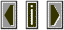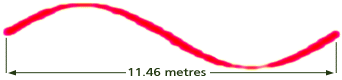WavelengthThe physical distance in space covered by one complete cycle of a periodic waveform as it passes through some medium such as air.

 Wavelength for sound propagating in air can be easily calculated using the following equation:  Wavelength = Speed of Sound/Frequency  For example, if we want to calculate the wavelength of one cycle of a 30 Hz tone we would evaluate the following equation:  344 metres / 30 Hz = 11.46 metresor
1130 feet / 30 Hz = 37.66 feet